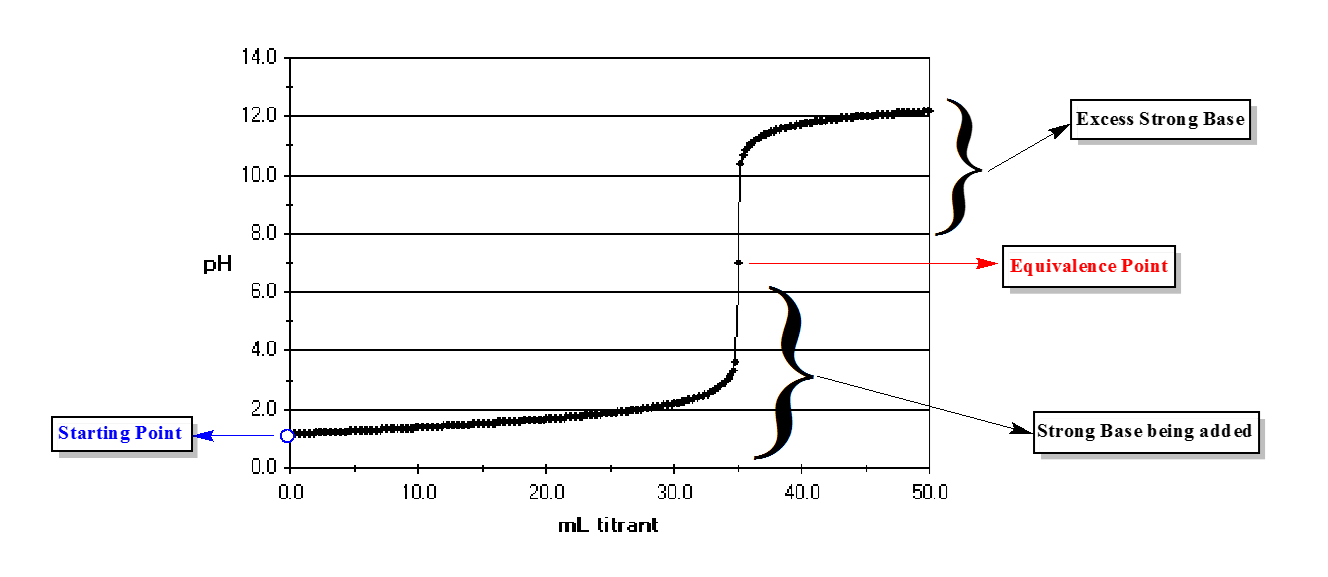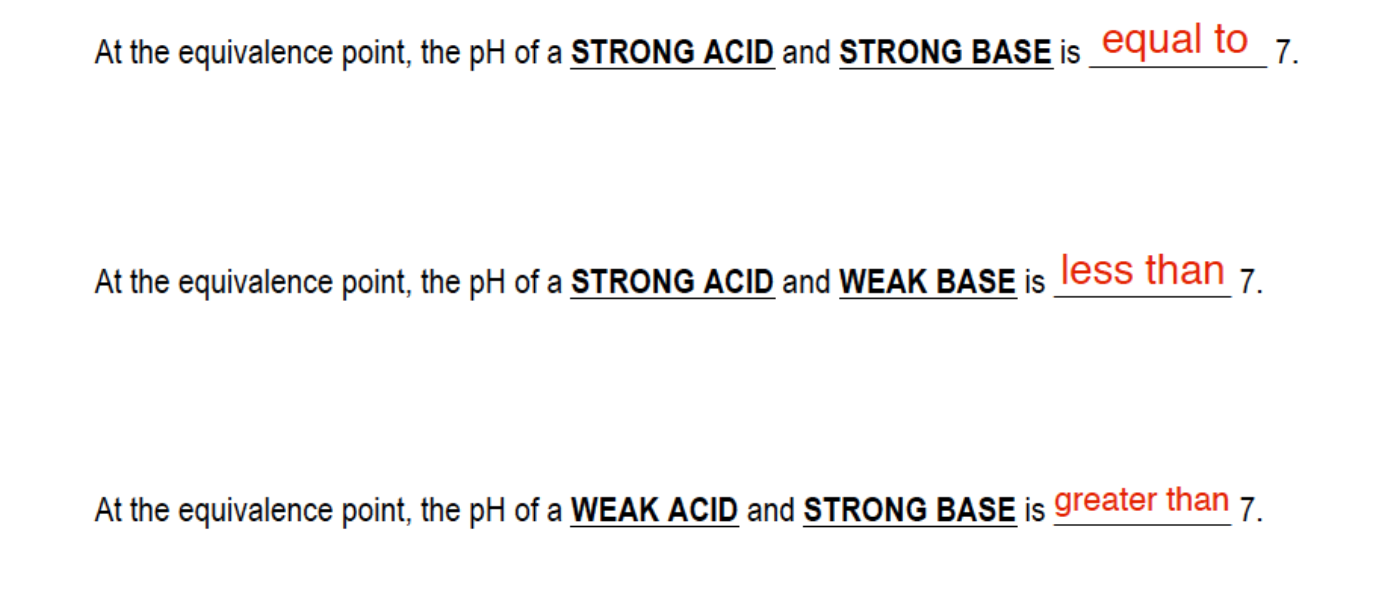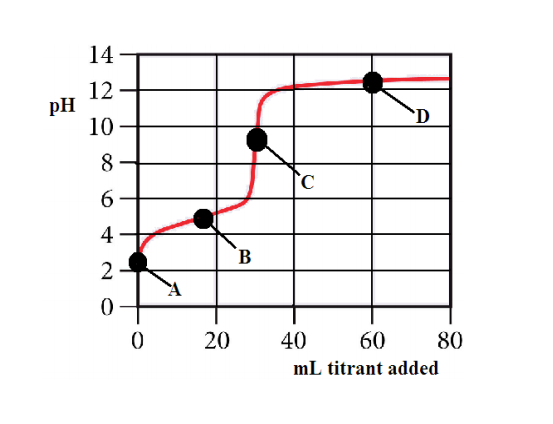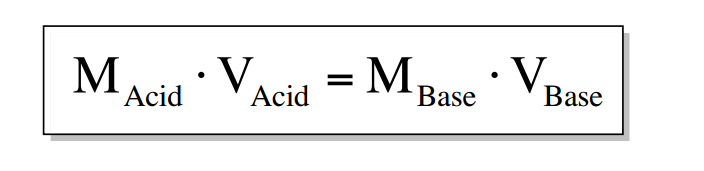Ch.16 - Aqueous Equilibrium WorksheetSee all chapters
 Ch.1 - Intro to General Chemistry 2hrs & 53mins 0% complete Worksheet Ch.2 - Atoms & Elements 2hrs & 49mins 0% complete Worksheet Ch.3 - Chemical Reactions 3hrs & 25mins 0% complete Worksheet BONUS: Lab Techniques and Procedures 1hr & 38mins 0% complete Worksheet BONUS: Mathematical Operations and Functions 47mins 0% complete Worksheet Ch.4 - Chemical Quantities & Aqueous Reactions 3hrs & 30mins 0% complete Worksheet Ch.5 - Gases 3hrs & 47mins 0% complete Worksheet Ch.6 - Thermochemistry 2hrs & 28mins 0% complete Worksheet Ch.7 - Quantum Mechanics 2hrs & 35mins 0% complete Worksheet Ch.8 - Periodic Properties of the Elements 1hr & 57mins 0% complete Worksheet Ch.9 - Bonding & Molecular Structure 2hrs & 5mins 0% complete Worksheet Ch.10 - Molecular Shapes & Valence Bond Theory 1hr & 31mins 0% complete Worksheet Ch.11 - Liquids, Solids & Intermolecular Forces 3hrs & 40mins 0% complete Worksheet Ch.12 - Solutions 2hrs & 17mins 0% complete Worksheet Ch.13 - Chemical Kinetics 2hrs & 22mins 0% complete Worksheet Ch.14 - Chemical Equilibrium 2hrs & 26mins 0% complete Worksheet Ch.15 - Acid and Base Equilibrium 4hrs & 42mins 0% complete Worksheet Ch.16 - Aqueous Equilibrium 3hrs & 48mins 0% complete Worksheet Ch. 17 - Chemical Thermodynamics 1hr & 44mins 0% complete Worksheet Ch.18 - Electrochemistry 2hrs & 58mins 0% complete Worksheet Ch.19 - Nuclear Chemistry 1hr & 33mins 0% complete Worksheet Ch.20 - Organic Chemistry 3hrs 0% complete Worksheet Ch.22 - Chemistry of the Nonmetals 2hrs & 1min 0% complete Worksheet Ch.23 - Transition Metals and Coordination Compounds 1hr & 54mins 0% complete Worksheet

# Acid and Base Titration Curves

See all sections
###### Titration Curves

Titration curves help us to understand the neutralization occurring between an acid and base in order to identify the equivalence point.

###### Equivalence Point

The equivalence point is when the moles of acid and base titrating are equal in amount.

Concept #1: Understanding an Acid–Base Titration Curve.

Transcript

Hey guys, in this new video, we're going to take a look at acid and base titration curves. Let's take a look at this image. We're going to say here the shape of a titration curve involving an acid and a base makes it possible for us to identify the equivalence point. Now, we're going to say the equivalence point is where we have equal amounts of acid and base together. It's when we have equal moles of both. We'll have equal moles of acid and base. That is called or equivalence point. They basically neutralize each other because they're equal in amount. If we take a look at this curve, we can say here that what looks like we’re beginning around a pH of 1. That pH is very, very low. That would indicate that we're dealing with a strong acid initially. Take a look. Overtime we’re adding more ml of a titrate.
Basically what's happening is we have a jar or a beaker of acid and we're dropping, drop by drop, some base. We’re saying that the strong acid is being titrated by base. The base is our titrate. We're saying over time we can see that the pH is gradually increasing. Then all of a sudden, boom it goes straight up and there’s a huge change in the pH. We're going to say because around this volume where we see the big jump in our pH, this represents the equivalence volume of our base. It looks like once we get to 35 ml, we've reached the equivalence amount of mls of the base we need to get to the equivalence point. It would shoot straight up. This is the steepest climb. The biggest change in pH happens around the equivalence point. We're going to say halfway up this increase, we have this dot right here. That dot right there represents our equivalence point. At that exact moment, we have equal amounts of acid and base together. We're going to say here it looks like the pH is 7 exactly at the equivalence point. This is our equivalence point right here. We're going to say here because the equivalence point is exactly at 7, a pH of 7, this tells us that a strong acid is being titrated by a strong base. Because when they're titrating each other, and when we get to the equivalence point of a strong acid and a strong base together, the pH is always equal to 7 under normal conditions.
What we're going to say here, we see that it keeps climbing, the pH keeps climbing. After the equivalence point, we have what's called an excess of strong base. Remember, above 7 you’re basic. Once we get past this point right here, this equivalence point, all the acid is gone so all we have now is just additional base being added to our beaker of solution. This is exactly how it works. This would represent a pH titration curve of a strong acid and a strong base being mixed together.
We’ll learn later on that we don't only have this type of titration. We could titrate a strong base with a strong acid. We're starting out with strong base. It means we’re going to start off fairly high, maybe around 13 and we're going to add strong base. Overtime, the base will decrease and decrease. We’d say here, this red curve represents the titrating of a strong base with a strong acid. It would also give us a pH of equal to 7, around the equivalence point. So, these are two strong species titrating each other. We’re also going to learn later on that we have a strong species titrating a weak species. As a result, the pH at the equivalence point will be greater than 7 or less than 7.

In a typical titration we begin with an acid or base and slowly add either a Strong Acid or Strong Base.Concept #2: The Equivalence Point.

Transcript

If we take a look down below this graph, we’ve already answered this first part. We’re going to say at the equivalence point, the pH of a strong acid and a strong base is equal to 7. If we’re looking at a strong acid and a weak base, just remember, whoever is weak will dictate what kind of solution you have at the end. Since we're dealing with a strong acid, the acid is stronger now than the base. It's going to say since I’m stronger, I’m going to want my solution at the end to be acidic. Anytime you're titrating a weak acid and a strong base together, at the equivalence point the pH is going to be less than 7. It's going to be acidic. It’s acidic because the acid is stronger. Whoever is stronger will tell us what kind of solution we have. In the first example, they both were strong. We wind up with a draw, which means neutral that's why the pH is equal to 7.
For the last scenario, we have a weak acid and a strong base. Now the base is stronger so it's going to dictate what kind of solution we have? Because we're dealing with a strong base, it’s going to want to have a basic solution. The solution has to have a pH greater than 7 at the equivalence point. Just remember these fundamentals. As we start to do more acid and base titration questions, keep this in mind as we face every type of question we're going to see. Just remember, the equivalence point is when we have equal moles of acid and base. Depending on what we're titrating, whether they both are strong, or one is strong or weak, the equivalence point could have a pH equal to 7, less than 7 or greater than 7.

Depending on the types of acids and bases mixing, at the equivalence point, the pH can be less than, greater than or equal to 7.Example #1: The following questions refer to the titration curve given below.

1) The titration curve shows the titration of

a) a strong acid with a strong base    b) a weak acid with a strong base    c) a strong base with a strong acid    d) a weak base with a strong acid

2) Which point on the titration curve represents a region where a buffer solution has formed?

a) point A      b) point B      c) point C      d) point D

3) Which point on the titration curve represents the equivalence point?

a) point A      b) point B      c) point C      d) point D

4) Which of the following would be the best indicator to use in the titration?

a) erythrosin B, pKa=2.9    b) methyl blue, pKa=5.4    c) bromthymol blue, pKa= 6.8    d) o-cresonphthalein, pKa=9.0An indicator represents a weak acid or base in low concentration that changes color in an acid base titration. An indicator shows us the location of our endpoint, which is located near our equivalence point.

Example #2:  The acid form of an indicator is red and its anion is blue. The Ka value for this indicator is 10 -9. What will be the approximate pH range over which this indicator changes color?
a) 3-5        b) 4-6        c) 5-7        d) 8-10        e) 9-11

An indicator, like a buffer, has an optimal range in which it works most effectively:

pka +/- 1.

Practice: What will be the color of the indicator in the above question in a solution that has a pH of 6?

Example #3: Consider the titration of 100.0 mL of 0.016 M H2SO4 with 0.400 M NaOH at the equivalence point. How many many milliliters of 0.400 M NaOH are required to reach the equivalence point?

If at the equivalence point, the moles of acid equal the moles of base and moles equal molarity multiplied by liters then at the equivalence point we can use the formula:Example #4: Consider the titration of 40.0 mL of 0.0800 M HCl with 0.0160 M Ca(NH2)2.

a) How many milliliters of 0.0160 M Ca(NH2)2 are required to reach the equivalence point?

When dealing with the equivalence point make sure you correctly calculate the molarity for both the acid and base.

Example #5: Consider the titration of 40.0 mL of 0.0800 M HCl with 0.0160 M Ca(NH2)2.

b) What is the pH of this solution?

Practice: Consider the titration of 60.0 mL of 0.200 M H3PO3 solution with 0.350 M potassium hydroxide, KOH solution. How many milliliters of base would be required to reach each of its equivalence points?